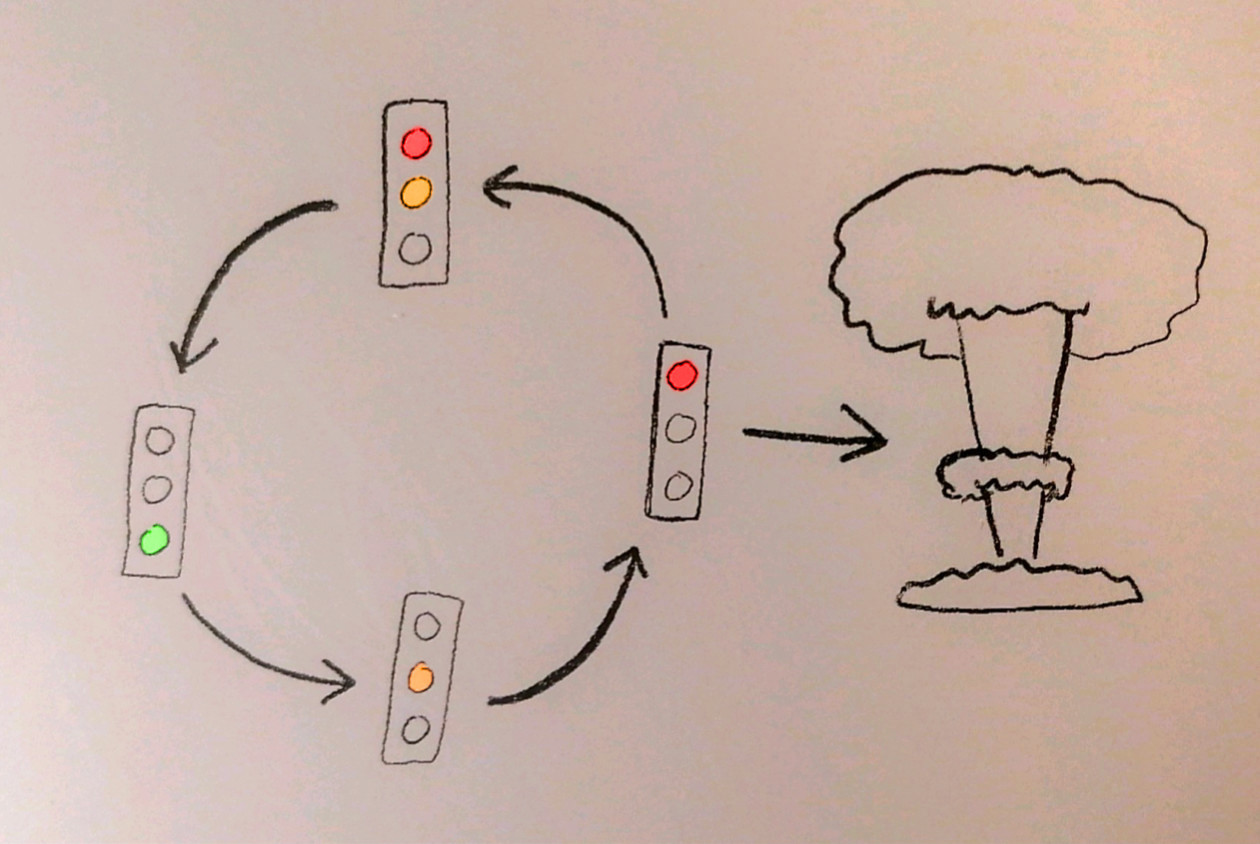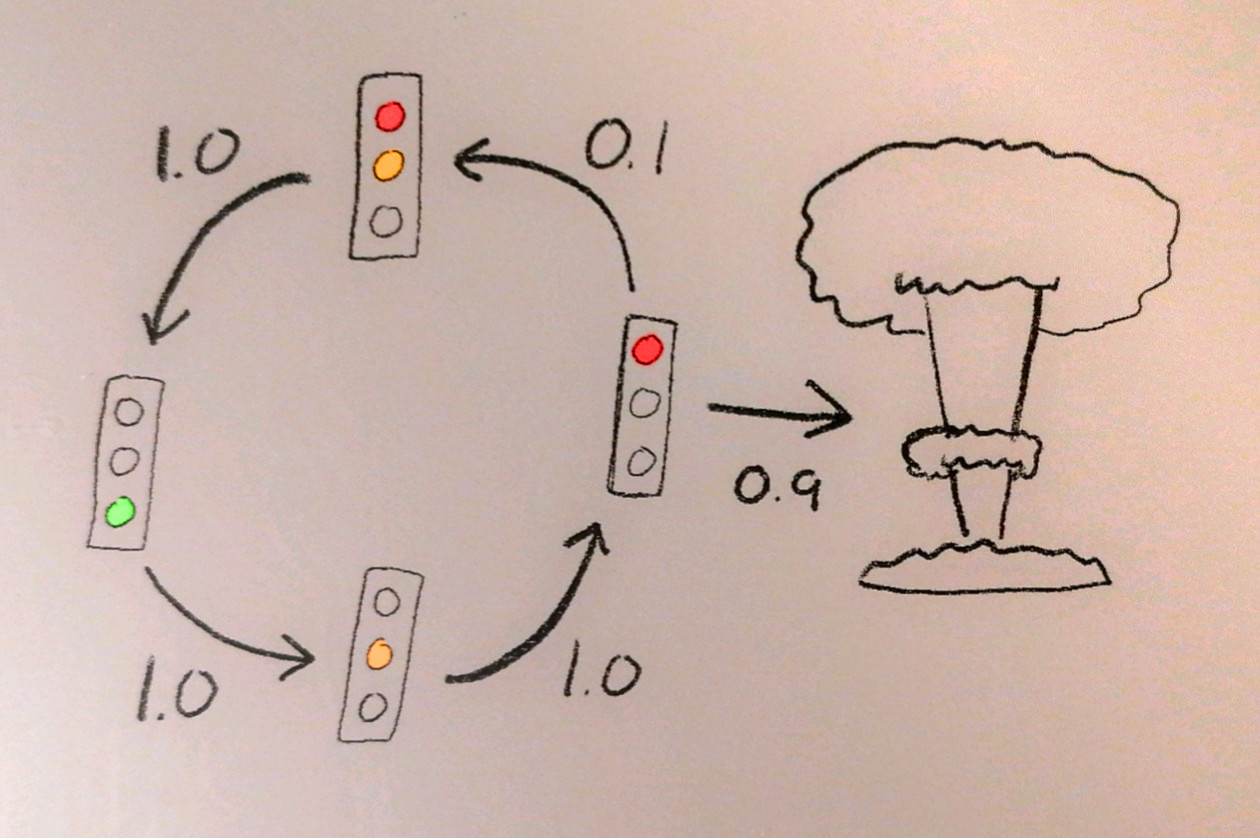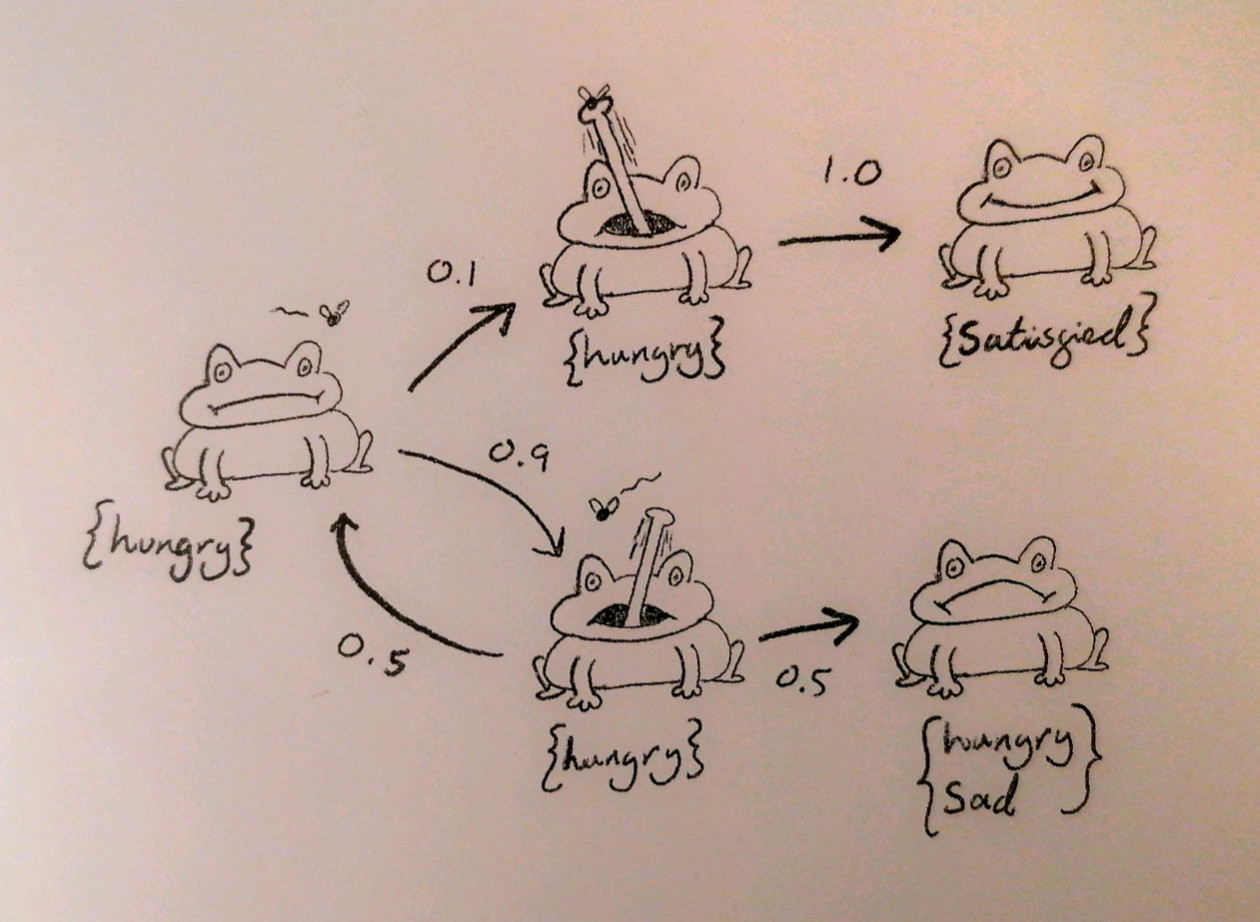# Finite Automata

Modelling real-world systems can be done with something called finite automata, a mathematical object in which we have a collection of labelled states, with transitions between them. For example, below is an automaton representing a UK traffic light, labelled with the colour the light is in a given state, with arrows representing the transitions between the states. Note that we’re modelling with discrete time-steps rather than continuous time. The labelling of states is done with so-called ‘atomic propositions’, these are basically low-level properties that we know hold in a given state.We are allowed to have multiple outgoing transitions from a state, in which case the choice is non-deterministic. This allows us to model uncertain real-world behaviour, such as the fact that when the traffic light is red there is a non-zero probability that it will explode!So, while this tells us which transitions could possibly be taken, it says nothing about the probability of those transitions. We can extend our definition of an automaton so that each transition has a probability of being taken. For this to make sense, the probabilities of all of a node’s outgoing edges must sum to one. This new type of automaton is called a Markov chain or Markov process.The probability of taking a particular path through the automaton, i.e. a particular sequence of connected states, is the product of the probabilities of the connecting transitions that we take along the way.

# Probabilistic Computation Tree Logic (PCTL)

While this structure allows us to model real-world systems with quantified uncertainty, we would then like to be able to answer complex probabilistic questions about these systems. For example, when considering a communication protocol, we may want to verify that “There is at least a 95% probability that within 5 time-steps of sending a message, we receive an acknowledgement”. To formally express statements like these, we use a logic called Probabilistic Computation Tree Logic (PCTL). This logic has a branching model of time where in a given state there may be multiple outgoing paths into future states, creating a tree structure that expands outwards as time progresses.

PCTL has the standard logical operators like negation, conjuction, implication, etc., but the fundamental temporal building block of PCTL is the “until” operator:

$f_1 \; U_{\leq t}^{\geq p} \; f_2$

which says that with a probability of at least $$p$$, within $$t$$ time-steps the sub-formula $$f_2$$ will hold, and the sub-formula $$f_1$$ will hold continuously up until this point.

Let us create a model of a hungry frog who wants to eat a fly, but is very inaccurate. The frog begins in its initial state as being hungry. It tries to eat the fly, with a 10% probability of success and a 90% probability of failure. If it succeeds then the frog is satisfied, if it fails then the frog is still hungry, but is now sad as well. In this state, the fly has a 50% probability of making its escape, and so the frog stays permanently hungry and sad. Otherwise the frog gets another chance at eating it.We may want to claim that there is at least a 10% probability that within 10 time-steps, the frog is satisfied (i.e. it has eaten the fly), which we would formulate as:

$true \; U_{\leq 10}^{\geq 0.1} \; \texttt{satisfied}$

(The formula $$true$$ holds vacuously in all states, which allows us to make no claims about the path taken to get to satisfaction.)

It’s all well and good to claim that this formula holds in our model, but how do we validate our claim? This is where model checking comes in, which is a way to automatically verify whether a temporal logic formula holds in a given model.

# Model Checking

🚧🚧 Section under construction! 🚧🚧

We solve our problem from the bottom-up, finding solutions for each of the constituent parts of our formula, and then combining these solutions together in a compositional way so that we eventually get the answer for the full formula.

This algorithm operates by ‘labelling’ states with sub-formulae, with the labelling of a complex formulae being dependent on which states are labelled with its constituent sub-formulae. As an example, let’s check the formula

$\texttt{P} \; U_{\leq 3}^{\geq p} \; \texttt{Q} \wedge \texttt{R}$

in the model

IMAGE

We start with the left sub-formula $$\texttt{P}$$, which holds in all states that have the atomic property $$\texttt{P}$$, so we label those states accordingly. We then move onto the right sub-formulae, individually labelling the states which are labelled with $$\texttt{Q}$$ and those that are labelled with $$\texttt{R}$$. To do the labelling of $$\texttt{Q} \wedge \texttt{R}$$, we can label the states which are already labelled with both $$\texttt{Q}$$ and $$\texttt{R}$$. This compositional labelling works similarly for all of the basic operators. You may have noticed that so far this technique doesn’t allow the labelling of one state to affect another state: they are completely independent. In order to model temporal properties then, we must extend the algorithm.

As an introduction to this idea we can first consider the case where $$p=1$$ for the temporal statement above, i.e. that the statement is guaranteed to hold, rather than just having some possibility of holding. This is saying that from a given starting state, within three time-steps all paths will end in a state where $$\texttt{Q} \wedge \texttt{R}$$ holds, and that $$\texttt{P}$$ will hold in all states before this point.

We can compute this recursively in a top-down manner, starting in the starting state and recursing on each outgoing edge to the neighbouring states. In each state, if the state is labelled with $$\texttt{Q} \wedge \texttt{R}$$ then we are done for this particular path and know that it satisfies the whole formula. Otherwise, if the state is not labelled with $$\texttt{P}$$ or if the path length has reached its maximum value, then we fail this path. In fact, because $$p=1$$, this allows us to conclude that the formula does not hold for the starting state, since this path is a counterexample.

As this is a property of states, and we may compose together multiple temporal operators, we must perform this state exploration algorithm independently from every state, so that at the end each state is either labelled with $$\texttt{P} \; U_{\leq 3}^{\geq p} \; \texttt{Q} \wedge \texttt{R}$$ or not.

We now cover the case of model checking when $$0 < p < 1$$. We take advantage of the probabilistic nature of transitions in the Markov chain. Instead of our recursive algorithm only considering whether transitions can or cannot happen, we instead compute the cumulative probability of sets of paths that satisfy the formula, with the following general recurrence equation for $$f_1 \; U_{\leq t}^{\geq p} \; f_2$$:

\begin{align*} P(t,s) = \; & \text{if } f_2 \in label(s) \text{ then } 1 \\ & \text{else if } f_1 \notin label(s) \text{ or } t = 0 \text{ then } 0 \\ & \text{else } \sum_{s'\in S} T(s,s') \times P(t-1, s') \end{align*}

As above, we compute $$P(t,s)$$ for all states $$s$$, and we then label $$s$$ with the formula only if $$P(t,s) \geq p$$, in other words we have concluded that starting from $$s$$, the formula will hold within $$t$$ time-steps with a probability greater than or equal to $$p$$.